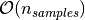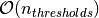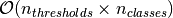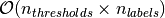Shortcuts

# AUROC¶

## Module Interface¶

class torchmetrics.AUROC(task: Literal['binary', 'multiclass', 'multilabel'], thresholds: = None, num_classes: = None, num_labels: = None, average: Optional[Literal['macro', 'weighted', 'none']] = 'macro', max_fpr: = None, ignore_index: = None, validate_args: bool = True, **kwargs: Any)[source]

Compute Area Under the Receiver Operating Characteristic Curve (ROC AUC). The AUROC score summarizes the ROC curve into an single number that describes the performance of a model for multiple thresholds at the same time. Notably, an AUROC score of 1 is a perfect score and an AUROC score of 0.5 corresponds to random guessing.

This module is a simple wrapper to get the task specific versions of this metric, which is done by setting the task argument to either 'binary', 'multiclass' or multilabel. See the documentation of BinaryAUROC, MulticlassAUROC and MultilabelAUROC for the specific details of each argument influence and examples.

Legacy Example:
>>> preds = torch.tensor([0.13, 0.26, 0.08, 0.19, 0.34])
>>> target = torch.tensor([0, 0, 1, 1, 1])
>>> auroc(preds, target)
tensor(0.5000)

>>> preds = torch.tensor([[0.90, 0.05, 0.05],
...                       [0.05, 0.90, 0.05],
...                       [0.05, 0.05, 0.90],
...                       [0.85, 0.05, 0.10],
...                       [0.10, 0.10, 0.80]])
>>> target = torch.tensor([0, 1, 1, 2, 2])
>>> auroc(preds, target)
tensor(0.7778)


### BinaryAUROC¶

class torchmetrics.classification.BinaryAUROC(max_fpr=None, thresholds=None, ignore_index=None, validate_args=True, **kwargs)[source]

Compute Area Under the Receiver Operating Characteristic Curve (ROC AUC) for binary tasks. The AUROC score summarizes the ROC curve into an single number that describes the performance of a model for multiple thresholds at the same time. Notably, an AUROC score of 1 is a perfect score and an AUROC score of 0.5 corresponds to random guessing.

As input to forward and update the metric accepts the following input:

• preds (Tensor): A float tensor of shape (N, ...) containing probabilities or logits for each observation. If preds has values outside [0,1] range we consider the input to be logits and will auto apply sigmoid per element.

• target (Tensor): An int tensor of shape (N, ...) containing ground truth labels, and therefore only contain {0,1} values (except if ignore_index is specified). The value 1 always encodes the positive class.

As output to forward and compute the metric returns the following output:

Additional dimension ... will be flattened into the batch dimension.

The implementation both supports calculating the metric in a non-binned but accurate version and a binned version that is less accurate but more memory efficient. Setting the thresholds argument to None will activate the non-binned version that uses memory of sizewhereas setting the thresholds argument to either an integer, list or a 1d tensor will use a binned version that uses memory of size(constant memory).

Parameters
• max_fpr (Optional[float]) – If not None, calculates standardized partial AUC over the range [0, max_fpr].

• Can be one of:

• If set to None, will use a non-binned approach where thresholds are dynamically calculated from all the data. Most accurate but also most memory consuming approach.

• If set to an int (larger than 1), will use that number of thresholds linearly spaced from 0 to 1 as bins for the calculation.

• If set to an list of floats, will use the indicated thresholds in the list as bins for the calculation

• If set to an 1d tensor of floats, will use the indicated thresholds in the tensor as bins for the calculation.

• validate_args (bool) – bool indicating if input arguments and tensors should be validated for correctness. Set to False for faster computations.

• kwargs (Any) – Additional keyword arguments, see Advanced metric settings for more info.

Example

>>> from torchmetrics.classification import BinaryAUROC
>>> preds = torch.tensor([0, 0.5, 0.7, 0.8])
>>> target = torch.tensor([0, 1, 1, 0])
>>> metric = BinaryAUROC(thresholds=None)
>>> metric(preds, target)
tensor(0.5000)
>>> b_auroc = BinaryAUROC(thresholds=5)
>>> b_auroc(preds, target)
tensor(0.5000)


Initializes internal Module state, shared by both nn.Module and ScriptModule.

### MulticlassAUROC¶

class torchmetrics.classification.MulticlassAUROC(num_classes, average='macro', thresholds=None, ignore_index=None, validate_args=True, **kwargs)[source]

Compute Area Under the Receiver Operating Characteristic Curve (ROC AUC) for multiclass tasks. The AUROC score summarizes the ROC curve into an single number that describes the performance of a model for multiple thresholds at the same time. Notably, an AUROC score of 1 is a perfect score and an AUROC score of 0.5 corresponds to random guessing.

As input to forward and update the metric accepts the following input:

• preds (Tensor): A float tensor of shape (N, C, ...) containing probabilities or logits for each observation. If preds has values outside [0,1] range we consider the input to be logits and will auto apply softmax per sample.

• target (Tensor): An int tensor of shape (N, ...) containing ground truth labels, and therefore only contain values in the [0, n_classes-1] range (except if ignore_index is specified).

As output to forward and compute the metric returns the following output:

• mc_auroc (Tensor): If average=None|”none” then a 1d tensor of shape (n_classes, ) will be returned with auroc score per class. If average=”macro”|”weighted” then a single scalar is returned.

Additional dimension ... will be flattened into the batch dimension.

The implementation both supports calculating the metric in a non-binned but accurate version and a binned version that is less accurate but more memory efficient. Setting the thresholds argument to None will activate the non-binned version that uses memory of sizewhereas setting the thresholds argument to either an integer, list or a 1d tensor will use a binned version that uses memory of size(constant memory).

Parameters
• num_classes (int) – Integer specifing the number of classes

• average (Optional[Literal[‘macro’, ‘weighted’, ‘none’]]) –

Defines the reduction that is applied over classes. Should be one of the following:

• macro: Calculate score for each class and average them

• weighted: Calculates score for each class and computes weighted average using their support

• "none" or None: Calculates score for each class and applies no reduction

• Can be one of:

• If set to None, will use a non-binned approach where thresholds are dynamically calculated from all the data. Most accurate but also most memory consuming approach.

• If set to an int (larger than 1), will use that number of thresholds linearly spaced from 0 to 1 as bins for the calculation.

• If set to an list of floats, will use the indicated thresholds in the list as bins for the calculation

• If set to an 1d tensor of floats, will use the indicated thresholds in the tensor as bins for the calculation.

• validate_args (bool) – bool indicating if input arguments and tensors should be validated for correctness. Set to False for faster computations.

• kwargs (Any) – Additional keyword arguments, see Advanced metric settings for more info.

Example

>>> from torchmetrics.classification import MulticlassAUROC
>>> preds = torch.tensor([[0.75, 0.05, 0.05, 0.05, 0.05],
...                       [0.05, 0.75, 0.05, 0.05, 0.05],
...                       [0.05, 0.05, 0.75, 0.05, 0.05],
...                       [0.05, 0.05, 0.05, 0.75, 0.05]])
>>> target = torch.tensor([0, 1, 3, 2])
>>> metric = MulticlassAUROC(num_classes=5, average="macro", thresholds=None)
>>> metric(preds, target)
tensor(0.5333)
>>> mc_auroc = MulticlassAUROC(num_classes=5, average=None, thresholds=None)
>>> mc_auroc(preds, target)
tensor([1.0000, 1.0000, 0.3333, 0.3333, 0.0000])
>>> mc_auroc = MulticlassAUROC(num_classes=5, average="macro", thresholds=5)
>>> mc_auroc(preds, target)
tensor(0.5333)
>>> mc_auroc = MulticlassAUROC(num_classes=5, average=None, thresholds=5)
>>> mc_auroc(preds, target)
tensor([1.0000, 1.0000, 0.3333, 0.3333, 0.0000])


Initializes internal Module state, shared by both nn.Module and ScriptModule.

### MultilabelAUROC¶

class torchmetrics.classification.MultilabelAUROC(num_labels, average='macro', thresholds=None, ignore_index=None, validate_args=True, **kwargs)[source]

Compute Area Under the Receiver Operating Characteristic Curve (ROC AUC) for multilabel tasks. The AUROC score summarizes the ROC curve into an single number that describes the performance of a model for multiple thresholds at the same time. Notably, an AUROC score of 1 is a perfect score and an AUROC score of 0.5 corresponds to random guessing.

As input to forward and update the metric accepts the following input:

• preds (Tensor): A float tensor of shape (N, C, ...) containing probabilities or logits for each observation. If preds has values outside [0,1] range we consider the input to be logits and will auto apply sigmoid per element.

• target (Tensor): An int tensor of shape (N, C, ...) containing ground truth labels, and therefore only contain {0,1} values (except if ignore_index is specified).

As output to forward and compute the metric returns the following output:

• ml_auroc (Tensor): If average=None|”none” then a 1d tensor of shape (n_classes, ) will be returned with auroc score per class. If average=”micro|macro”|”weighted” then a single scalar is returned.

Additional dimension ... will be flattened into the batch dimension.

The implementation both supports calculating the metric in a non-binned but accurate version and a binned version that is less accurate but more memory efficient. Setting the thresholds argument to None will activate the non-binned version that uses memory of sizewhereas setting the thresholds argument to either an integer, list or a 1d tensor will use a binned version that uses memory of size(constant memory).

Parameters
• num_labels (int) – Integer specifing the number of labels

• average (Optional[Literal[‘micro’, ‘macro’, ‘weighted’, ‘none’]]) –

Defines the reduction that is applied over labels. Should be one of the following:

• micro: Sum score over all labels

• macro: Calculate score for each label and average them

• weighted: Calculates score for each label and computes weighted average using their support

• "none" or None: Calculates score for each label and applies no reduction

• Can be one of:

• If set to None, will use a non-binned approach where thresholds are dynamically calculated from all the data. Most accurate but also most memory consuming approach.

• If set to an int (larger than 1), will use that number of thresholds linearly spaced from 0 to 1 as bins for the calculation.

• If set to an list of floats, will use the indicated thresholds in the list as bins for the calculation

• If set to an 1d tensor of floats, will use the indicated thresholds in the tensor as bins for the calculation.

• validate_args (bool) – bool indicating if input arguments and tensors should be validated for correctness. Set to False for faster computations.

• kwargs (Any) – Additional keyword arguments, see Advanced metric settings for more info.

Example

>>> from torchmetrics.classification import MultilabelAUROC
>>> preds = torch.tensor([[0.75, 0.05, 0.35],
...                       [0.45, 0.75, 0.05],
...                       [0.05, 0.55, 0.75],
...                       [0.05, 0.65, 0.05]])
>>> target = torch.tensor([[1, 0, 1],
...                        [0, 0, 0],
...                        [0, 1, 1],
...                        [1, 1, 1]])
>>> ml_auroc = MultilabelAUROC(num_labels=3, average="macro", thresholds=None)
>>> ml_auroc(preds, target)
tensor(0.6528)
>>> ml_auroc = MultilabelAUROC(num_labels=3, average=None, thresholds=None)
>>> ml_auroc(preds, target)
tensor([0.6250, 0.5000, 0.8333])
>>> ml_auroc = MultilabelAUROC(num_labels=3, average="macro", thresholds=5)
>>> ml_auroc(preds, target)
tensor(0.6528)
>>> ml_auroc = MultilabelAUROC(num_labels=3, average=None, thresholds=5)
>>> ml_auroc(preds, target)
tensor([0.6250, 0.5000, 0.8333])


Initializes internal Module state, shared by both nn.Module and ScriptModule.

## Functional Interface¶

torchmetrics.functional.auroc(preds, target, task, thresholds=None, num_classes=None, num_labels=None, average='macro', max_fpr=None, ignore_index=None, validate_args=True)[source]

Compute Area Under the Receiver Operating Characteristic Curve (ROC AUC). The AUROC score summarizes the ROC curve into an single number that describes the performance of a model for multiple thresholds at the same time. Notably, an AUROC score of 1 is a perfect score and an AUROC score of 0.5 corresponds to random guessing.

This function is a simple wrapper to get the task specific versions of this metric, which is done by setting the task argument to either 'binary', 'multiclass' or multilabel. See the documentation of binary_auroc(), multiclass_auroc() and multilabel_auroc() for the specific details of each argument influence and examples.

Legacy Example:
>>> preds = torch.tensor([0.13, 0.26, 0.08, 0.19, 0.34])
>>> target = torch.tensor([0, 0, 1, 1, 1])
tensor(0.5000)

>>> preds = torch.tensor([[0.90, 0.05, 0.05],
...                       [0.05, 0.90, 0.05],
...                       [0.05, 0.05, 0.90],
...                       [0.85, 0.05, 0.10],
...                       [0.10, 0.10, 0.80]])
>>> target = torch.tensor([0, 1, 1, 2, 2])
tensor(0.7778)

Return type

### binary_auroc¶

torchmetrics.functional.classification.binary_auroc(preds, target, max_fpr=None, thresholds=None, ignore_index=None, validate_args=True)[source]

Compute Area Under the Receiver Operating Characteristic Curve (ROC AUC) for binary tasks. The AUROC score summarizes the ROC curve into an single number that describes the performance of a model for multiple thresholds at the same time. Notably, an AUROC score of 1 is a perfect score and an AUROC score of 0.5 corresponds to random guessing.

Accepts the following input tensors:

• preds (float tensor): (N, ...). Preds should be a tensor containing probabilities or logits for each observation. If preds has values outside [0,1] range we consider the input to be logits and will auto apply sigmoid per element.

• target (int tensor): (N, ...). Target should be a tensor containing ground truth labels, and therefore only contain {0,1} values (except if ignore_index is specified). The value 1 always encodes the positive class.

Additional dimension ... will be flattened into the batch dimension.

The implementation both supports calculating the metric in a non-binned but accurate version and a binned version that is less accurate but more memory efficient. Setting the thresholds argument to None will activate the non-binned version that uses memory of sizewhereas setting the thresholds argument to either an integer, list or a 1d tensor will use a binned version that uses memory of size(constant memory).

Parameters
• preds (Tensor) – Tensor with predictions

• target (Tensor) – Tensor with true labels

• max_fpr (Optional[float]) – If not None, calculates standardized partial AUC over the range [0, max_fpr].

• Can be one of:

• If set to None, will use a non-binned approach where thresholds are dynamically calculated from all the data. Most accurate but also most memory consuming approach.

• If set to an int (larger than 1), will use that number of thresholds linearly spaced from 0 to 1 as bins for the calculation.

• If set to an list of floats, will use the indicated thresholds in the list as bins for the calculation

• If set to an 1d tensor of floats, will use the indicated thresholds in the tensor as bins for the calculation.

• validate_args (bool) – bool indicating if input arguments and tensors should be validated for correctness. Set to False for faster computations.

Return type
Returns

A single scalar with the auroc score

Example

>>> from torchmetrics.functional.classification import binary_auroc
>>> preds = torch.tensor([0, 0.5, 0.7, 0.8])
>>> target = torch.tensor([0, 1, 1, 0])
>>> binary_auroc(preds, target, thresholds=None)
tensor(0.5000)
>>> binary_auroc(preds, target, thresholds=5)
tensor(0.5000)


### multiclass_auroc¶

torchmetrics.functional.classification.multiclass_auroc(preds, target, num_classes, average='macro', thresholds=None, ignore_index=None, validate_args=True)[source]

Compute Area Under the Receiver Operating Characteristic Curve (ROC AUC) for multiclass tasks. The AUROC score summarizes the ROC curve into an single number that describes the performance of a model for multiple thresholds at the same time. Notably, an AUROC score of 1 is a perfect score and an AUROC score of 0.5 corresponds to random guessing.

Accepts the following input tensors:

• preds (float tensor): (N, C, ...). Preds should be a tensor containing probabilities or logits for each observation. If preds has values outside [0,1] range we consider the input to be logits and will auto apply softmax per sample.

• target (int tensor): (N, ...). Target should be a tensor containing ground truth labels, and therefore only contain values in the [0, n_classes-1] range (except if ignore_index is specified).

Additional dimension ... will be flattened into the batch dimension.

The implementation both supports calculating the metric in a non-binned but accurate version and a binned version that is less accurate but more memory efficient. Setting the thresholds argument to None will activate the non-binned version that uses memory of sizewhereas setting the thresholds argument to either an integer, list or a 1d tensor will use a binned version that uses memory of size(constant memory).

Parameters
• preds (Tensor) – Tensor with predictions

• target (Tensor) – Tensor with true labels

• num_classes (int) – Integer specifing the number of classes

• average (Optional[Literal[‘macro’, ‘weighted’, ‘none’]]) –

Defines the reduction that is applied over classes. Should be one of the following:

• macro: Calculate score for each class and average them

• weighted: Calculates score for each class and computes weighted average using their support

• "none" or None: Calculates score for each class and applies no reduction

• Can be one of:

• If set to None, will use a non-binned approach where thresholds are dynamically calculated from all the data. Most accurate but also most memory consuming approach.

• If set to an int (larger than 1), will use that number of thresholds linearly spaced from 0 to 1 as bins for the calculation.

• If set to an list of floats, will use the indicated thresholds in the list as bins for the calculation

• If set to an 1d tensor of floats, will use the indicated thresholds in the tensor as bins for the calculation.

• validate_args (bool) – bool indicating if input arguments and tensors should be validated for correctness. Set to False for faster computations.

Return type

Tensor

Returns

If average=None|”none” then a 1d tensor of shape (n_classes, ) will be returned with auroc score per class. If average=”macro”|”weighted” then a single scalar is returned.

Example

>>> from torchmetrics.functional.classification import multiclass_auroc
>>> preds = torch.tensor([[0.75, 0.05, 0.05, 0.05, 0.05],
...                       [0.05, 0.75, 0.05, 0.05, 0.05],
...                       [0.05, 0.05, 0.75, 0.05, 0.05],
...                       [0.05, 0.05, 0.05, 0.75, 0.05]])
>>> target = torch.tensor([0, 1, 3, 2])
>>> multiclass_auroc(preds, target, num_classes=5, average="macro", thresholds=None)
tensor(0.5333)
>>> multiclass_auroc(preds, target, num_classes=5, average=None, thresholds=None)
tensor([1.0000, 1.0000, 0.3333, 0.3333, 0.0000])
>>> multiclass_auroc(preds, target, num_classes=5, average="macro", thresholds=5)
tensor(0.5333)
>>> multiclass_auroc(preds, target, num_classes=5, average=None, thresholds=5)
tensor([1.0000, 1.0000, 0.3333, 0.3333, 0.0000])


### multilabel_auroc¶

torchmetrics.functional.classification.multilabel_auroc(preds, target, num_labels, average='macro', thresholds=None, ignore_index=None, validate_args=True)[source]

Compute Area Under the Receiver Operating Characteristic Curve (ROC AUC) for multilabel tasks. The AUROC score summarizes the ROC curve into an single number that describes the performance of a model for multiple thresholds at the same time. Notably, an AUROC score of 1 is a perfect score and an AUROC score of 0.5 corresponds to random guessing.

Accepts the following input tensors:

• preds (float tensor): (N, C, ...). Preds should be a tensor containing probabilities or logits for each observation. If preds has values outside [0,1] range we consider the input to be logits and will auto apply sigmoid per element.

• target (int tensor): (N, C, ...). Target should be a tensor containing ground truth labels, and therefore only contain {0,1} values (except if ignore_index is specified).

Additional dimension ... will be flattened into the batch dimension.

The implementation both supports calculating the metric in a non-binned but accurate version and a binned version that is less accurate but more memory efficient. Setting the thresholds argument to None will activate the non-binned version that uses memory of sizewhereas setting the thresholds argument to either an integer, list or a 1d tensor will use a binned version that uses memory of size(constant memory).

Parameters
• preds (Tensor) – Tensor with predictions

• target (Tensor) – Tensor with true labels

• num_labels (int) – Integer specifing the number of labels

• average (Optional[Literal[‘micro’, ‘macro’, ‘weighted’, ‘none’]]) –

Defines the reduction that is applied over labels. Should be one of the following:

• micro: Sum score over all labels

• macro: Calculate score for each label and average them

• weighted: Calculates score for each label and computes weighted average using their support

• "none" or None: Calculates score for each label and applies no reduction

• Can be one of:

• If set to None, will use a non-binned approach where thresholds are dynamically calculated from all the data. Most accurate but also most memory consuming approach.

• If set to an int (larger than 1), will use that number of thresholds linearly spaced from 0 to 1 as bins for the calculation.

• If set to an list of floats, will use the indicated thresholds in the list as bins for the calculation

• If set to an 1d tensor of floats, will use the indicated thresholds in the tensor as bins for the calculation.

• validate_args (bool) – bool indicating if input arguments and tensors should be validated for correctness. Set to False for faster computations.

Return type
Returns

If average=None|”none” then a 1d tensor of shape (n_classes, ) will be returned with auroc score per class. If average=”micro|macro”|”weighted” then a single scalar is returned.

Example

>>> from torchmetrics.functional.classification import multilabel_auroc
>>> preds = torch.tensor([[0.75, 0.05, 0.35],
...                       [0.45, 0.75, 0.05],
...                       [0.05, 0.55, 0.75],
...                       [0.05, 0.65, 0.05]])
>>> target = torch.tensor([[1, 0, 1],
...                        [0, 0, 0],
...                        [0, 1, 1],
...                        [1, 1, 1]])
>>> multilabel_auroc(preds, target, num_labels=3, average="macro", thresholds=None)
tensor(0.6528)
>>> multilabel_auroc(preds, target, num_labels=3, average=None, thresholds=None)
tensor([0.6250, 0.5000, 0.8333])
>>> multilabel_auroc(preds, target, num_labels=3, average="macro", thresholds=5)
tensor(0.6528)
>>> multilabel_auroc(preds, target, num_labels=3, average=None, thresholds=5)
tensor([0.6250, 0.5000, 0.8333])


© Copyright Copyright (c) 2020-2023, Lightning-AI et al... Revision 6853c553.

Built with Sphinx using a theme provided by Read the Docs.
Versions
latest
stable
v0.11.2
v0.11.1
v0.11.0
v0.10.3
v0.10.2
v0.10.1
v0.10.0
v0.9.3
v0.9.2
v0.9.1
v0.9.0
v0.8.2
v0.8.1
v0.8.0
v0.7.3
v0.7.2
v0.7.1
v0.7.0
v0.6.2
v0.6.1
v0.6.0
v0.5.1
v0.5.0
v0.4.1
v0.4.0
v0.3.2
v0.3.1
v0.3.0
v0.2.0
v0.1.0